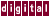## 7.3.3 Loop Peeling

In cases where an array is used to simulate a cylindrical coordinate system, where the left edge of the array must be adjacent to its right edge, a "wraparound variable" is often used, as follows:

```jm1 = n;
for ( j=0; j<n; j++ ) {
b[j] = (a[j] + a[jm1]) / 2;
jm1 = j;
}
```

In the first iteration, `jm1` is `n` . In all iterations except for `j=0` , the value of `jm1` is `j-1` . Thus, `jm1` is an induction variable for the loop after the first iteration.

By peeling off the first iteration of the loop, the `jm1` induction variable can be exploited, as follows:

```if (n >= 1) {
b = (a + a[n]) / 2;
for ( j = 1; j<n; j++ ) {
b[j] = (a[j] + a[j-1]) / 2;
}
}
```

KAP may peel off several iterations in cases where multiple wraparound variables exist.

Previous Page Next Page Contents Index
Command-Line Qualifiers